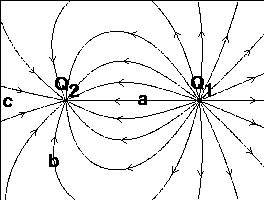# Problem: The figure shows the E-field in the plane of two-point charges.True or False.1) Q1 and Q2 have the same sign.2) A + charge at c would accelerate to the right.3) The magnitude of E is the same at a and b.4) A − charge at a would accelerate to the left.5) Q1 is positive.6) The size of Q1 is larger than that of Q2.

###### FREE Expert Solution

To determine whether the statements are true, let's review the properties of electric field:

• Electric field lines point away from a positive charge and towards a negative charge.
• The direction of the electric field is given by a force on positive test charge.
• The more the number of field lines or greater number density of electric field lines, the greater the magnitude of electric field or charge.
• Like charges repel while unlike charges attract.
92% (15 ratings)###### Problem DetailsThe figure shows the E-field in the plane of two-point charges.
True or False.
1) Q1 and Q2 have the same sign.
2) A + charge at c would accelerate to the right.
3) The magnitude of E is the same at a and b.
4) A − charge at a would accelerate to the left.
5) Q1 is positive.
6) The size of Q1 is larger than that of Q2.